Updating search results...

# 6 Results

View
Selected filters: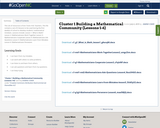Conditional Remix & Share Permitted
CC BY-NC-SA
Rating
0.0 stars

This set of resources is from Tools 4 NC Teachers.

This file contains a set of 6 lessons to be used during the first week(s) of school to develop students' mathematical mindsets. Lessons include:
Lesson 1: What is Math?
Lesson 2: Mathematicians Work Together
Lesson 3: Mathematicians Cooperate
Lesson 5: Mathematicians Learn from Mistakes
Lesson 6: Mathematicians Persevere

Subject:
Mathematics
Material Type:
Lesson
Lesson Plan
11/06/2019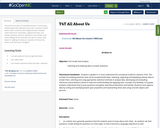Conditional Remix & Share Permitted
CC BY-NC-SA
Rating
0.0 stars

This resource is part of Tools4NCTeachers.

In this lesson, students generate "getting to know you" questions, which lead to both numerical and categorical data. Students collect data from classmates, organize the data, and answer questions about it. Questions may include: How many siblings do you have? Do you like ice cream.

Have ideas for "getting to know you" questions? Remix this lesson, and share your thoughts!

Subject:
Mathematics
Material Type:
Activity/Lab
Lesson
Lesson Plan
11/06/2019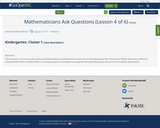Conditional Remix & Share Permitted
CC BY-NC-SA
Rating
0.0 stars

This resource is part of Tools4NCTeachers.

This is lesson 4 of 6 lessons focused around developing a mathematical community at the beginning of the school year. While this lesson addresses standards NC.K.MD.1 and NC.K.MD.2, its primary goal is for students to recognize that mathematicians ask questions to gather information.

Subject:
Mathematics
Material Type:
Lesson Plan
11/06/2019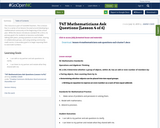Conditional Remix & Share Permitted
CC BY-NC-SA
Rating
0.0 stars

This resource is part of Tools4NCTeachers.

This is lesson four in a series of six lessons focused around developing a mathematical community at the beginning of the school year. While this lesson introduces standard NC.2.OA.3, its primary goal is for students to become comfortable talking with peers, posing questions to each other, sharing in collaborative groups, and using existing community math norms. A secondary goal is to begin exposing them to even/odd numbers.

Subject:
Mathematics
Material Type:
Lesson
Lesson Plan
11/06/2019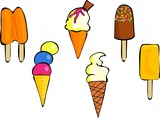Unrestricted Use
CC BY
Rating
0.0 stars

This resource is from Tools4NCTeachers.In this lesson, students represent and interpret three categories of data in charts and tables.&nbsp; They analyze the data to answer compare questions about how many more are in one category than another.&nbsp; The lesson may be modified to integrate or emphasize questions about how many fewer.&nbsp; Samples of student work and student recording sheets are included.Remix this lesson to include extension activites or related math stations.&nbsp; Sharing is caring!

Subject:
Mathematics
Material Type:
Lesson
Lesson Plan
Author:
DAWNE COKER
06/14/2020Unrestricted Use
CC BY
Rating
0.0 stars

This resource is from Tools4NCTeachers.&nbsp;&nbsp;This lesson will involve interviewing classmates about where they would like to spend their summer vacation.&nbsp; Their choices will be the beach, mountains, time with a relative, or camping.&nbsp; They will create a picture graph to represent the data collected.This lesson comes with printable materials and clipart for creating a class graph.

Subject:
Mathematics
Material Type:
Activity/Lab
Lesson
Lesson Plan
Author:
DAWNE COKER